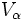# Manifold over a pseudogroup

## Definition

### Given data

A pseudogroup$G$ acting on a topological space$X$.

### Definition part

A topological space$M$ is termed a$G$-manifold if there is an open cover$U_\alpha$ of$M$ such that$U_\alpha$ are isomorphic to open sets$V_\alpha$ in$X$, and such that the transition functions are elements within$G$.

The underlying space$X$ here is termed the model space for the$G$-manifold structure.

## Examples

### Topological manifold

Further information: topological manifold

A topological manifold is a manifold over the pseudogroup of all homeomorphisms between open sets, with the model space being$\R^n$.

### Differential manifold

Further information: differential manifold

A differential manifold is a manifold over the pseudogroup of all diffeomorphisms between open sets, with the model space being$\R^n$.

### Real-analytic manifold

Further information: real-analytic manifold

A real-analytic manifold is a manifold over the pseudogroup of all real-analytic maps between open sets in$\R^n$, with the model space being$\R^n$.

### Measured manifold

Further information: measured manifold

A measured manifold is a manifold over the pseudogroup of all volume-preserving diffeomorphisms between open sets, with the model space being$\R^n$.